### Home > A2C > Chapter 2 > Lesson 2.1.3 > Problem2-36

2-36.
1. DeShawna and her team gathered data for their ball and recorded it in the table shown at right. Homework Help ✎

Drop Height

Rebound Height

150 cm

124 cm

70 cm

58.5 cm

120 cm

99.5 cm

100 cm

82.6 cm

110 cm

92 cm

40 cm

33.4 cm

1. What is the rebound ratio for their ball?

2. Predict how high DeShawna's ball will rebound if it is dropped from 3 meters.

3. Suppose the ball is dropped and you notice that its rebound height is 60 cm. From what height was the ball dropped?

4. Suppose the ball is dropped from a window 200 meters up the Empire State Building. What would you predict the rebound height to be after the first bounce?

5. How high would the ball rebound after the second bounce? After the third bounce?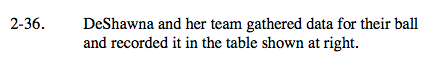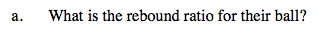Divide a rebound height by a drop height.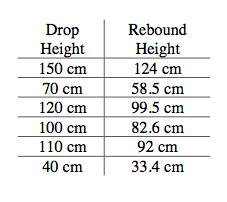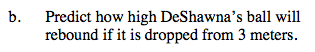Multiply the drop height by the ratio.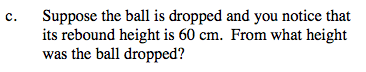Divide the drop height by the ratio.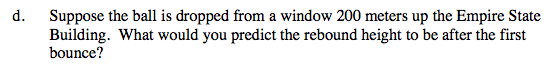See part (b).

The rebound height is about 166m.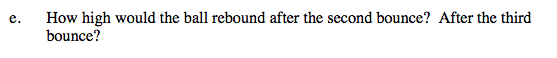Continue multiplying the drop height by the ratio to find the next rebound heights.